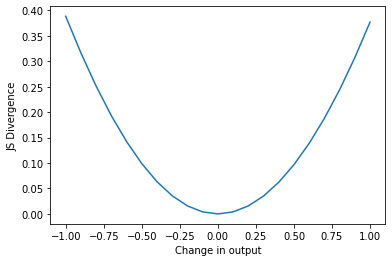import timm
import torch
import torch.nn.functional as F
from timm.loss import LabelSmoothingCrossEntropy, SoftTargetCrossEntropy
from timm.loss import JsdCrossEntropy
from timm.data.mixup import mixup_target
import matplotlib.pyplot as plt


Let's create a example of the output of a model, and our labels. Note we have 3 output predictions, but only 1 label.

output = F.one_hot(torch.tensor([0,9,0])).float()
labels=torch.tensor()


If we set label smoothing and alpha to 0, then we will have the regular cross_entropy loss, if we look only at the first element of our output and labels.

jsd = JsdCrossEntropy(smoothing=0,alpha=0)

jsd(output,labels)

tensor(1.4612)
base_loss = F.cross_entropy(output[0,None],labels[0,None])
base_loss

tensor(1.4612)
jsd = JsdCrossEntropy(num_splits=1,smoothing=0,alpha=0)


We can also change the number of splits,changing the size of each group. In Augmix this would equate to the number of transformation mixtures.

jsd = JsdCrossEntropy(num_splits=2,smoothing=0,alpha=0)
output = F.one_hot(torch.tensor([0,9,1,0])).float()
labels=torch.tensor([0,9])

jsd(output,labels),F.cross_entropy(output[[0,1]],labels)

(tensor(1.4612), tensor(1.4612))

By default we have 1 label for 3 predictions, this is a two part loss, and measures both cross entropy and jason-shannon divergence. Jason-shannon entropy does not need a label, instead measuring the how significantly different the 3 predictions are.

jsd = JsdCrossEntropy(smoothing=0)
output = F.one_hot(torch.tensor([0,0,0]),num_classes=10).float()

deltas = torch.cat((torch.zeros([2,10]),torch.tensor([[-1,1,0,0,0,0,0,0,0,0]])))*0.1
deltas

tensor([-0.1000,  0.1000,  0.0000,  0.0000,  0.0000,  0.0000,  0.0000,  0.0000,
0.0000,  0.0000])
deltas=(torch.arange(-10,11))[...,None,None]*deltas

losses = [jsd((output+delta),labels)-base_loss for delta in deltas]


The below graph shows how changes in one of the model's outputs(prediction), in a group, effects the Jason-Shannon Divergence.

plt.plot([ .1*i-1 for i in range(len(losses))],[loss for loss in losses])
plt.ylabel('JS Divergence')
plt.xlabel('Change in output')
plt.show()